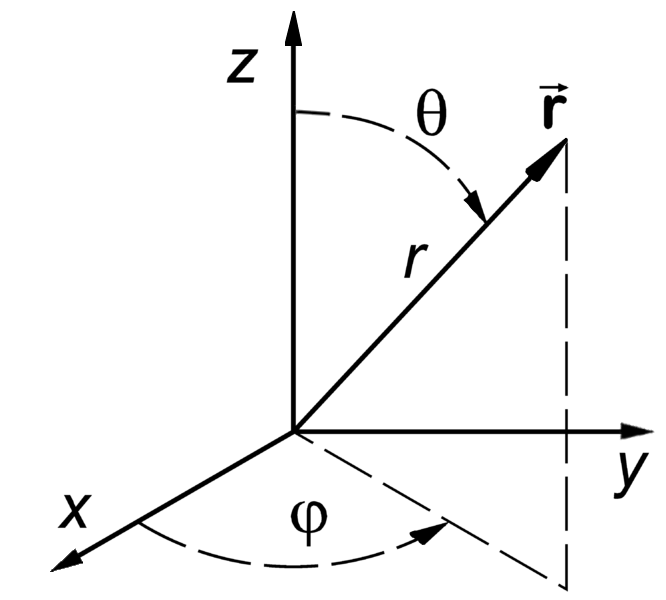# 2.2: The Schwarzschild Metric

•• Skidmore College
$$\newcommand{\vecs}{\overset { \rightharpoonup} {\mathbf{#1}} }$$ $$\newcommand{\vecd}{\overset{-\!-\!\rightharpoonup}{\vphantom{a}\smash {#1}}}$$$$\newcommand{\id}{\mathrm{id}}$$ $$\newcommand{\Span}{\mathrm{span}}$$ $$\newcommand{\kernel}{\mathrm{null}\,}$$ $$\newcommand{\range}{\mathrm{range}\,}$$ $$\newcommand{\RealPart}{\mathrm{Re}}$$ $$\newcommand{\ImaginaryPart}{\mathrm{Im}}$$ $$\newcommand{\Argument}{\mathrm{Arg}}$$ $$\newcommand{\norm}{\| #1 \|}$$ $$\newcommand{\inner}{\langle #1, #2 \rangle}$$ $$\newcommand{\Span}{\mathrm{span}}$$ $$\newcommand{\id}{\mathrm{id}}$$ $$\newcommand{\Span}{\mathrm{span}}$$ $$\newcommand{\kernel}{\mathrm{null}\,}$$ $$\newcommand{\range}{\mathrm{range}\,}$$ $$\newcommand{\RealPart}{\mathrm{Re}}$$ $$\newcommand{\ImaginaryPart}{\mathrm{Im}}$$ $$\newcommand{\Argument}{\mathrm{Arg}}$$ $$\newcommand{\norm}{\| #1 \|}$$ $$\newcommand{\inner}{\langle #1, #2 \rangle}$$ $$\newcommand{\Span}{\mathrm{span}}$$$$\newcommand{\AA}{\unicode[.8,0]{x212B}}$$

If you look out into space, you will find a lot of objects that are close to being spheres and that are very far away from other large objects. As an approximation, we can treat them as perfect spheres that are completely isolated. The most sensible coordinates to use for a sphere are spherical coordinates,

$x^\mu=\begin{pmatrix}t & r & \theta & \phi\end{pmatrix},$

where $$\phi$$ is the azimuthal angle (i.e. longitude, $$0\le \phi \le 2\pi$$), and $$\theta$$ is the polar angle (i.e. latitude, but measured from the north pole instead of the equator, $$0\le \theta \le \pi$$), as shown in Figure 2.2.1.Figure $$\PageIndex{1}$$: Spherical coordinates. (Image credit: Wikipedia)

Using this approximation in the EFE yields

$g_{\mu\nu}=\begin{pmatrix}1-\frac{2M}{r} & 0 & 0 & 0\\ 0 & -\frac{1}{1-\frac{2M}{r}} & 0 & 0\\ 0 & 0 & -r^2 & 0\\ 0 & 0 & 0 & -r^2\sin^2\theta\end{pmatrix},\label{eq:SchwarzschildMetric}$

where $$M$$ is the mass of the sphere. This is referred to as the Schwarzschild metric.

## Note

Even though this metric is different from the one in special relativity, the index notation rules still apply. That is, the index of a vector can be lowered using the metric (Box 2.2.1), the inverse metric $$g^{\mu\nu}$$ is defined by $$g_{\alpha\mu}g^{\mu\nu}=\mathbf{I}$$ (Box 2.2.2), and $$u_\mu u^\mu=1$$ (Box 2.2.3).

## Definition: Schwarzschild metric

The Schwarzschild metric is the metric for a (non-spinning) spherically symmetric mass in an otherwise empty spacetime.

The spacetime interval that goes along with Equation \ref{eq:SchwarzschildMetric} is

$d\tau^2=\left (1-\frac{2M}{r}\right)dt^2-\frac{1}{1-\frac{2M}{r}}dr^2-r^2d\theta^2-r^2\sin^2\theta d\phi^2.$

You may notice that, in order for the units to work out, the mass $$M$$ must have the same units as $$r$$! This is the result of using natural units, but this time setting Newton's Gravitational constant $$G=1$$, which results in the following conversion between mass and distance.

$1\text{ kg}=7.426 \times 10^{-28}\text{ m}\nonumber$

## Exercise $$\PageIndex{1}$$

What are the masses of the earth and sun in meters?

earth: $5.97\times 10^{24}\text{ kg}\times \frac{7.426\times 10^{-28}\text{ m}}{1\text{ kg}}=0.0044\text{ m}\nonumber$

sun: $1.99\times 10^{30}\text{ kg}\times \frac{7.426\times 10^{-28}\text{ m}}{1\text{ kg}}=1477\text{ m}\nonumber$

## Box $$\PageIndex{1}$$

Show that for a four-vector a, the vector with downstairs index using the Schwarschild metric is $$a_t=\left(1-\frac{2M}{r}\right)a^t$$, $$a_r=-\frac{1}{1-\frac{2M}{r}}a^r$$, $$a_\theta=-r^2a^\theta$$, and $$a_\phi=-r^2\sin^2\theta a^\phi$$.

## Box $$\PageIndex{2}$$

Show that the inverse Schwarzschild metric $$g^{\mu\nu}=\begin{pmatrix}\frac{1}{1-\frac{2M}{r}} & 0 & 0 & 0\\ 0 & -\left(1-\frac{2M}{r}\right) & 0 & 0\\ 0 & 0 & -\frac{1}{r^2} & 0\\ 0 & 0 & 0 & -\frac{1}{r^2\sin^2\theta}\end{pmatrix}$$.

## Box $$\PageIndex{3}$$

Using the Schwarzschild metric, show that $$u_\mu u^\mu=1$$.

This page titled 2.2: The Schwarzschild Metric is shared under a not declared license and was authored, remixed, and/or curated by Evan Halstead.